# Pretty scatter plots with ggplot2

May 15, 2017
By

Want to share your content on R-bloggers? click here if you have a blog, or here if you don't.

@drsimonj here to make pretty scatter plots of correlated variables with ggplot2!

We’ll learn how to create plots that look like this:## Data

In a data.frame `d`, we’ll simulate two correlated variables `a` and `b` of length `n`:

``````set.seed(170513)
n <- 200
d <- data.frame(a = rnorm(n))
d\$b <- .4 * (d\$a + rnorm(n))

#>            a           b
#> 1 -0.9279965 -0.03795339
#> 2  0.9133158  0.21116682
#> 3  1.4516084  0.69060249
#> 4  0.5264596  0.22471694
#> 5 -1.9412516 -1.70890512
#> 6  1.4198574  0.30805526
``````

## Basic scatter plot

Using ggplot2, the basic scatter plot (with `theme_minimal`) is created via:

``````library(ggplot2)

ggplot(d, aes(a, b)) +
geom_point() +
theme_minimal()
``````## Shape and size

There are many ways to tweak the `shape` and `size` of the points. Here’s the combination I settled on for this post:

``````ggplot(d, aes(a, b)) +
geom_point(shape = 16, size = 5) +
theme_minimal()
``````## Color

We want to color the points in a way that helps to visualise the correlation between them.

One option is to `color` by one of the variables. For example, color by `a` (and hide legend):

``````ggplot(d, aes(a, b, color = a)) +
geom_point(shape = 16, size = 5, show.legend = FALSE) +
theme_minimal()
``````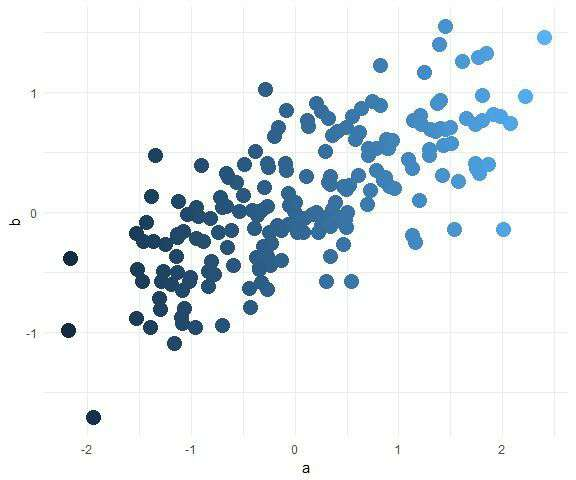Although it’s subtle in this plot, the problem is that the color is changing as the points go from left to right. Instead, we want the color to change in a direction that characterises the correlation – diagonally in this case.

To do this, we can color points by the first principal component. Add it to the data frame as a variable `pc` and use it to color like so:

``````d\$pc <- predict(prcomp(~a+b, d))[,1]

ggplot(d, aes(a, b, color = pc)) +
geom_point(shape = 16, size = 5, show.legend = FALSE) +
theme_minimal()
``````Now we can add color, let’s pick something nice with the help of the `scale_color_gradient` functions and some nice hex codes (check out color-hex for inspriation). For example:

``````ggplot(d, aes(a, b, color = pc)) +
geom_point(shape = 16, size = 5, show.legend = FALSE) +
theme_minimal() +
scale_color_gradient(low = "#0091ff", high = "#f0650e")
``````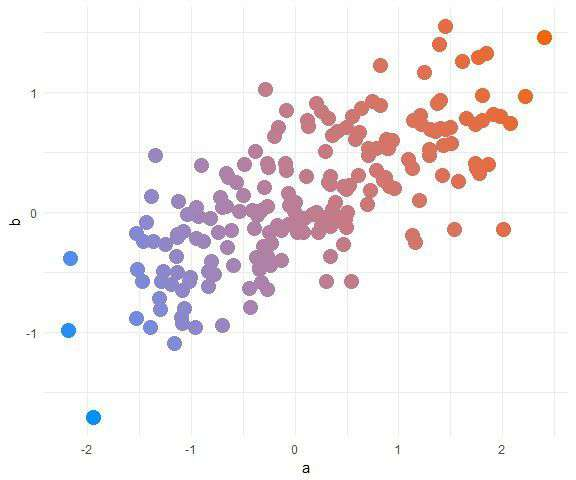## Transparency

Now it’s time to get rid of those offensive mushes by adjusting the transparency with `alpha`.

We could adjust it to be the same for every point:

``````ggplot(d, aes(a, b, color = pc)) +
geom_point(shape = 16, size = 5, show.legend = FALSE, alpha = .4) +
theme_minimal() +
scale_color_gradient(low = "#0091ff", high = "#f0650e")
``````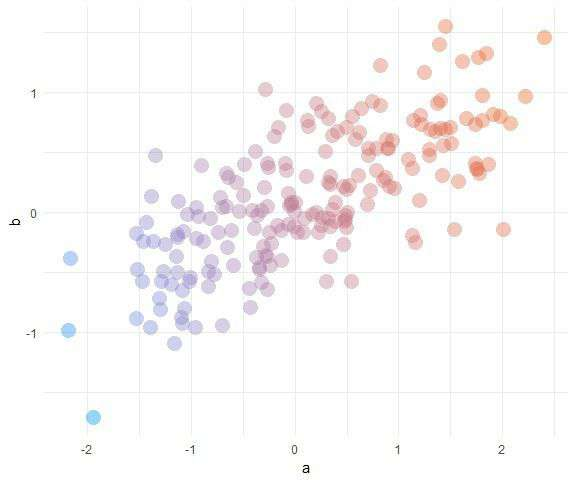This is fine most of the time. However, what if you have many points? Let’s try with 5,000 points:

``````# Simulate data
set.seed(170513)
n <- 5000
d <- data.frame(a = rnorm(n))
d\$b <- .4 * (d\$a + rnorm(n))

# Compute first principal component
d\$pc <- predict(prcomp(~a+b, d))[,1]

# Plot
ggplot(d, aes(a, b, color = pc)) +
geom_point(shape = 16, size = 5, show.legend = FALSE, alpha = .4) +
theme_minimal() +
scale_color_gradient(low = "#0091ff", high = "#f0650e")
``````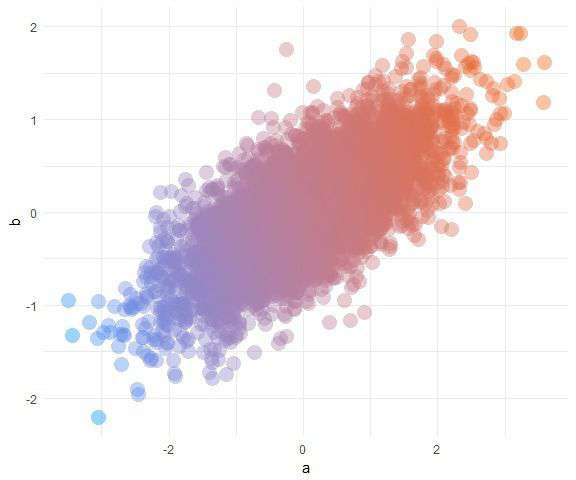We’ve got another big mush. What if we take `alpha` down really low to .05?

``````ggplot(d, aes(a, b, color = pc)) +
geom_point(shape = 16, size = 5, show.legend = FALSE, alpha = .05) +
theme_minimal() +
scale_color_gradient(low = "#0091ff", high = "#f0650e")
``````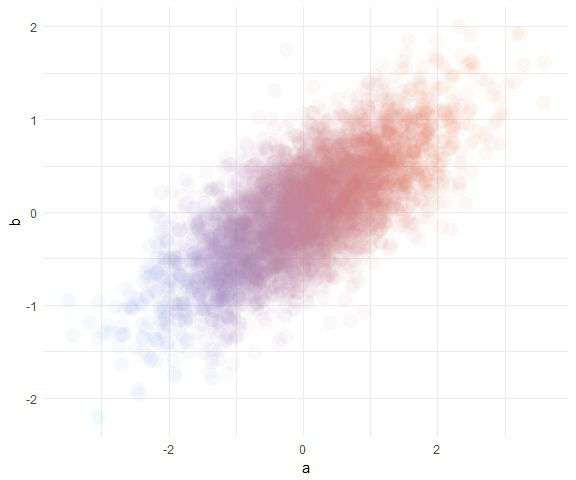Better, except it’s now hard to see extreme points that are alone in space.

To solve this, we’ll map `alpha` to the inverse point density. That is, turn down `alpha` wherever there are lots of points! The trick is to use bivariate density, which can be added as follows:

``````# Add bivariate density for each point
d\$density <- fields::interp.surface(
MASS::kde2d(d\$a, d\$b), d[,c("a", "b")])
``````

Now plot with `alpha` mapped to `1/density`:

``````ggplot(d, aes(a, b, color = pc, alpha = 1/density)) +
geom_point(shape = 16, size = 5, show.legend = FALSE) +
theme_minimal() +
scale_color_gradient(low = "#0091ff", high = "#f0650e")
``````You can see that distant points are now too vibrant. Our final fix is to use `scale_alpha` to tweak the alpha range. By default, this range is 0 to 1, making the most distant points have an alpha close to 1. Let’s restrict it to something better:

``````ggplot(d, aes(a, b, color = pc, alpha = 1/density)) +
geom_point(shape = 16, size = 5, show.legend = FALSE) +
theme_minimal() +
scale_color_gradient(low = "#0091ff", high = "#f0650e") +
scale_alpha(range = c(.05, .25))
``````Much better! No more mushy patches or lost points.

## Bringing it together

Here’s a complete example with new data and colors:

``````# Simulate data
set.seed(170513)
n <- 2000
d <- data.frame(a = rnorm(n))
d\$b <- -(d\$a + rnorm(n, sd = 2))

d\$pc <- predict(prcomp(~a+b, d))[,1]

# Add density for each point
d\$density <- fields::interp.surface(
MASS::kde2d(d\$a, d\$b), d[,c("a", "b")])

# Plot
ggplot(d, aes(a, b, color = pc, alpha = 1/density)) +
geom_point(shape = 16, size = 5, show.legend = FALSE) +
theme_minimal() +
scale_color_gradient(low = "#32aeff", high = "#f2aeff") +
scale_alpha(range = c(.25, .6))
``````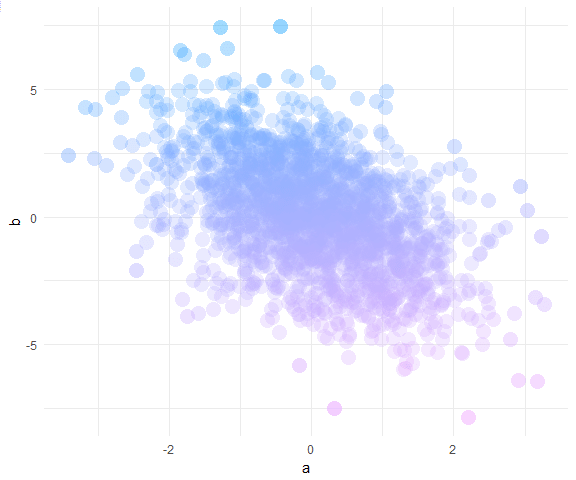## Sign off

Thanks for reading and I hope this was useful for you.

For updates of recent blog posts, follow @drsimonj on Twitter, or email me at [email protected] to get in touch.

If you’d like the code that produced this blog, check out the blogR GitHub repository.

R-bloggers.com offers daily e-mail updates about R news and tutorials about learning R and many other topics. Click here if you're looking to post or find an R/data-science job.
Want to share your content on R-bloggers? click here if you have a blog, or here if you don't.